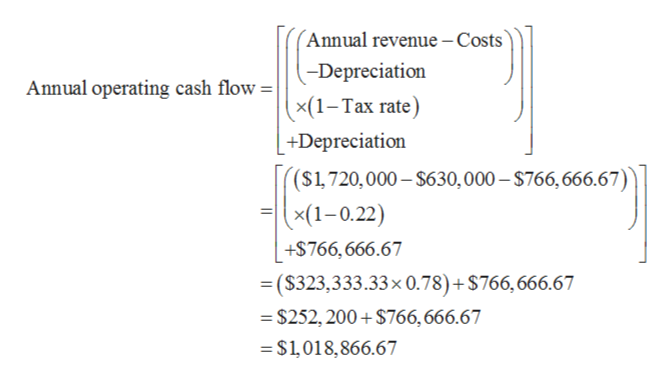# Down Under Boomerang, Inc., is considering a new 3-year expansion project that requires an initial fixed asset investment of \$2.3 million. The fixed asset will be depreciated straight-line to zero over its 3-year tax life. The project is estimated to generate \$1,720,000 in annual sales, with costs of \$630,000. The project requires an initial investment in net working capital of \$270,000, and the fixed asset will have a market value of \$210,000 at the end of the project. a.If the tax rate is 22 percent, what is the project’s Year 0 net cash flow? Year 1? Year 2? Year 3? (Do not round intermediate calculations and enter your answers in dollars, not millions of dollars, e.g., 1,234,567. A negative answer should be indicated by a minus sign.)b.If the required return is 10 percent, what is the project's NPV? (Do not round intermediate calculations and round your answer to 2 decimal places, e.g., 32.16.)     a.  whar are Years 0,1,2,3 cash flow seperatelyb.  What is the NPV

Question
141 views
 Down Under Boomerang, Inc., is considering a new 3-year expansion project that requires an initial fixed asset investment of \$2.3 million. The fixed asset will be depreciated straight-line to zero over its 3-year tax life. The project is estimated to generate \$1,720,000 in annual sales, with costs of \$630,000. The project requires an initial investment in net working capital of \$270,000, and the fixed asset will have a market value of \$210,000 at the end of the project.

 a. If the tax rate is 22 percent, what is the project’s Year 0 net cash flow? Year 1? Year 2? Year 3? (Do not round intermediate calculations and enter your answers in dollars, not millions of dollars, e.g., 1,234,567. A negative answer should be indicated by a minus sign.) b. If the required return is 10 percent, what is the project's NPV? (Do not round intermediate calculations and round your answer to 2 decimal places, e.g., 32.16.)

a.  whar are Years 0,1,2,3 cash flow seperately

b.  What is the NPV

check_circle

star
star
star
star
star
1 Rating
Step 1

The initial investment cost of store is \$2,300,000.

According to straight line depreciation, the annual depreciation is calculated below:

Step 2

a.

Cash outflow at the beginning of project is calculated below:

Step 3

When calculating operating gross profit, depreciation is deducted from revenue which will decrease the cash flow. So, it is needed to add b...help_outlineImage Transcriptionclose(Annual revenue -Costs -Depreciation x(1-Tax rate) Annual operating cash flow = | +Depreciation (S1,720,000-\$630, 000-\$766,666.67) (1-0.22) +\$766,666.67 = (\$323,333.33 x 0.78) + \$766, 666.67 =\$252, 200+ \$766, 666.67 \$1,018,866.67 fullscreen

### Want to see the full answer?

See Solution

#### Want to see this answer and more?

Solutions are written by subject experts who are available 24/7. Questions are typically answered within 1 hour.*

See Solution
*Response times may vary by subject and question.
Tagged in